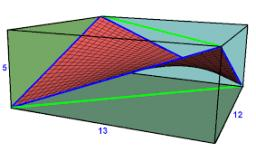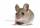# The rectangle

Determine the area of the rectangle where the length and width are in the ratio 5:2 and its length is 7.5 cm longer than its width. Determine also its length and its width.

a =  12.5 cm
b =  5 cm
S =  62.5 cm2

### Step-by-step explanation:

2a=5b
a = 7.5 + b

2•a=5•b
a = 7.5 + b

2a-5b = 0
a-b = 7.5

a = 25/2 = 12.5
b = 5

Our linear equations calculator calculates it.Did you find an error or inaccuracy? Feel free to write us. Thank you!Tips to related online calculators
Do you have a system of equations and looking for calculator system of linear equations?
Do you want to convert length units?

#### You need to know the following knowledge to solve this word math problem:

We encourage you to watch this tutorial video on this math problem:

## Related math problems and questions:

• The circumferenceThe circumference and width of the rectangle are in a ratio of 5: 1. its area is 216cm2. What is its length?
• RectanglePerimeter of rectangle is 48 cm. Calculate its dimensions if they are in the ratio 5:3 (width:height)
• RectangleThe width of the rectangle is 65% of its length. Perimeter of the rectangle is 132 cm. Determine the dimensions of the rectangle.
• The sidesThe sides of the rectangle are in a ratio of 3: 5 and its circumference measures 72 cm. Calculate: a) the size of both sides of the rectangle b) the area of the rectangle c) the length of the diagonals
• Rectangle - sides 4Perimeter of the rectangle is 72 cm. Calculate the length of the sides that are in the ratio 3:5.
• RectangleThe length of the rectangle is 12 cm greater than 3 times its width. What dimensions and area this rectangle has if ts circumference is 104 cm.
• RectangleFind the dimensions of the rectangle, whose perimeter is 108 cm and the length is 25% larger than the width.
• Dimensions of rectangleFind the dimensions of a rectangle given that its perimeter is 110 cm and its length is 1 cm more than twice its width.
• Rectangle - sidesWhat is the perimeter of a rectangle with area 266 cm2 if length of the shorter side is 5 cm shorter than the length of the longer side?
• Rectangles - sidesOne side of the rectangle is 10 cm longer than a second. Shortens longer side by 6 cm and extends shorter by 14 cm increases the rectangle area by 130 cm2. What are the dimensions of the original rectangle?
• RectangleThe perimeter of the rectangle is 22 cm and content area 30 cm2. Determine its dimensions, if the length of the sides of the rectangle in centimeters is expressed by integers.
• Similarity coefficientThe ratio of similarity of two equilateral triangles is 3.5 (ie 7:2). The length of the side of smaller triangle is 2.4 cm. Calculate the perimeter and area of ​​the larger triangle.
• RectangleThe length of the rectangle are in the ratio 5:12 and the circumference is 238 cm. Calculate the length of the diagonal and area of rectangle.
• Rectangle diagonalsIt is given a rectangle with an area of 24 cm2 a circumference of 20 cm. The length of one side is 2 cm larger than the length of the second side. Calculate the length of the diagonal. Length and width are yet expressed in natural numbers.
• ParallelogramThe perimeter of the parallelogram is 417 cm. The length of one side is 1.7-times longer than the length of the shorter side. What is the length of sides of a parallelogram?
• The sidesThe sides of a rectangle are in a ratio of 2:3, and its perimeter is 1 1/4 inches. What are the lengths of its side? Draw it.
• Rectangle from stringString 12m. Make rectangle when one side is two times longer than its width.